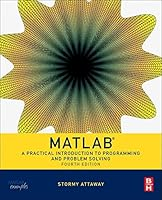# Matlab, 4th Edition: A Practical Introduction to Programming and Problem Solving## Book Description

: A Practical Introduction to Programming and Problem Solving, Fourth Edition, has been updated to reflect the functionality of the current version of MATLAB, including the new H2 system. It features new and revised end-of-chapter exercises, more engineering applications to help the reader learn this tool in context, and a new section on object-oriented programming in MATLAB. MATLAB has become the standard tool for solving and engineering problems due to its powerful built-in functions and its ability to program.

Assuming no knowledge of programming, this book guides the reader both programming and built-in functions to easily exploit MATLAB's extensive capabilities for tackling engineering problems. The book starts with programming concepts, such as variables, assignments, and selection statements, moves on to loops, and then solves problems using both the programming concept and the power of MATLAB. In-depth coverage is given to input/output, a topic fundamental to many engineering applications.

• Presents programming concepts and MATLAB built-in functions side-by-side
• Offers a systematic, step-by-step approach, building on concepts throughout the book and facilitating easier learning
• Includes sections on common pitfalls and programming guidelines to direct students toward best practices
• Combines basic programming concepts, built-in functions, and advanced topics for problem solving with MATLAB to make this book uniquely suitable for a wide range of courses teaching or using MATLAB across the curriculum

Part 1 Introduction To Programming Using MATLAB
Chapter 1 Introduction To MATLAB
Chapter 2 Vectors And Matrices
Chapter 3 Introduction To MATLAB Programming
Chapter 4 Selection Statements
Chapter 5 Loop Statements And Vectorizing
Chapter 6 MATLAB Programs
Chapter 7 String Manipulation
Chapter 8

Part 2 Advanced Topics For Problem Solving With MATLAB
Chapter 9 Advanced File Input And Output